﻿ 不同喷油策略对燃生物柴油船用柴油机燃烧和排放的影响
 舰船科学技术2020, Vol. 42Issue (8): 145-151    DOI: 10.3404/j.issn.1672-7649.2020.08.027PDF

1. 南通航运职业技术学院 轮机工程系，江苏 南通 226010;
2. 集美大学 轮机工程学院，福建 厦门 361021

Effect of different injection strategies on combustion and emission characteristics of a marine diesel engine fueled with biodiesel
YU Shi-yong1, LI Xiao-fei2
1. Marine Engineering Department, Nantong Shipping College, Nantong 226010, China;
2. School of Marine Engineering, College of Marine Engineering, Jimei University, Xiamen 361021, China
Abstract: Due to the advantages of renewable, non-toxic, degradable and sustainable, biodiesel has attracted more and more experts' attention. In order to analyze the influence of the characteristics of biodiesel and the injection strategy on the combustion and emission characteristics of diesel engine, a three-dimensional CFD simulation model is established in this paper, and the calculation is carried out by the coupling method of the CHEMKIN II code and the three-dimensional AVL fire. The calculation mechanism mainly considers 69 products and 204 chemical reactions of biodiesel saturation, and studies the influence of three different fuel injection strategies on diesel engine power, cylinder pressure, heat release rate, CO and NOx emissions under two conditions by using the verified simulation model.
Key words: AVL-fire     biodiesel     diesel engine     diesel engine emission
0 引　言

1 仿真模型的建立 1.1 数学模型

1）燃烧、排放和传热模型

 ${q_w} = \frac{{\rho {c_{p,F}}{u^ * }T\ln (\frac{T}{{{T_W}}}) - (2.1{y^ + } + 33.4)Gv/{u^ * }}}{{2.1\ln ({y^ + }) + 2.5}}\text{,}$ (1)
 ${y^ + } = \frac{{{u^ * } \times y}}{v}\text{,}$ (2)
 ${u^ * } = \sqrt {\frac{{{\tau _w}}}{\rho }} \text{。}$ (3)

 ${q_w} = \frac{{\rho {c_{p,F}}{u^ * }T\ln (\frac{T}{{{T_W}}})}}{{2.1\ln ({y^ + }) + 2.5}}\text{。}$ (4)

2）临界参数

 ${p_c} = M(0.339 + \sum {{\Delta _p}{)^{ - 2}}}\text{,}$ (5)
 ${T_c} = {T_b}\left[ {1 + {{\left( {1.242 + \sum {{\Delta _T}} } \right)}^{ - 1}}} \right]\text{。}$ (6)

 ${V_c} = 17.5 + \sum {{\Delta _V}}\text {,}$ (7)

3）蒸汽压

 ${p_{vp}}_r = {p_c}{e^{[{f^{(0)}}(T) + w{f^{(1)}}(T)]}}\text{,}$ (8)
 $\begin{split} {f^{(0)}}(T) = & 5.927\;14 - 6.096\;48\frac{{{T_c}}}{T} - \\ & 1.288\;62\ln \left( {\frac{T}{{{T_c}}}} \right) + 0.169\;347{\left( {\frac{T}{{{T_c}}}} \right)^6} \text{，} \end{split}$ (9)
 $\begin{split} {f^{(1)}}(T) = & 15.251\;8 - 15.687\;5\frac{{{T_c}}}{T} - \\ & 13.472\;1\ln \left( {\frac{T}{{{T_c}}}} \right) + 0.435\;77{\left( {\frac{T}{{{T_c}}}} \right)^6} \text{。} \end{split}$ (10)

4）液相密度

 $\phi = {(1 - T/{T_c})^{2/7}} - {(1 - {T_{r - R}})^{2/7}}\text{,}$ (11)
 ${V_s} = {V_{S - R}}Z_{RA}^\phi\text{,}$ (12)

 $\rho = \frac{{{\rho _R}}}{{Z_{RA}^\phi }}\text{,}$ (13)

5）汽化潜热

 $\Delta L = R{T_c}[7.08{(1 - \frac{{{T_c}}}{T})^{0.354}} + 10.95w{(1 - \frac{{{T_c}}}{T})^{0.456}}]\text{,}$ (14)

6）液相粘度

 $\ln \frac{{{\eta _L}}}{{{\rho _{20}}M}} = A + \frac{B}{T}\text{。}$ (15)

7）液相导热系数

 ${A_x} = \frac{{{A^*}T_b^\alpha }}{{{M^\beta }T_c^\gamma }}\text{,}$ (16)
 ${\lambda _L} = \displaystyle\frac{{{A_x}{{\left( {1 - \frac{{{T_c}}}{T}} \right)}^{0.38}}}}{{\left( {\displaystyle\frac{{{T_c}}}{T}} \right)_{}^{1/6}}}\text{。}$ (17)

8）气体扩散系数

 ${D_A} = A + B + C{T^2}\text{。}$ (18)

9）表面张力

 $\frac{\sigma }{{p_c^{2/3}T_c^{1/3}}} = \left( {0.132{\alpha _c} - 0.279} \right){(1 - {T_c}/T)^{11/9}}\text{,}$ (19)
 ${\alpha _c} = 0.907\;6\left[ {1 + \frac{{{T_{br}}\ln \left( {{p_c}/1.013\;25} \right)}}{{1 - {T_{br}}}}} \right]\text{。}$ (20)

1.2 AVL-Fire仿真模型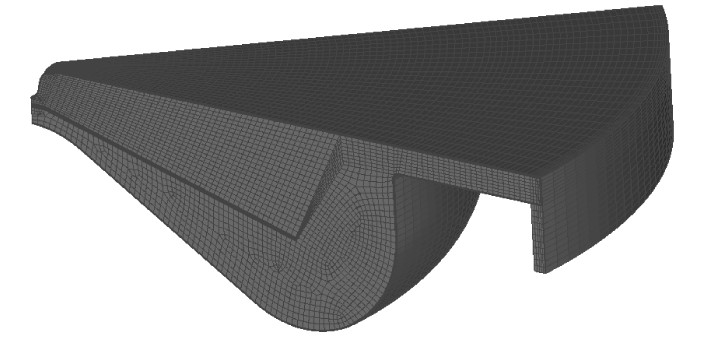图 1 上止点时45°燃烧室网格 Fig. 1 The 45° sector grid shown at TDC
1.3 仿真模型实验验证表 1 柴油机主要参数 Tab.1 Main parameters of diesel engine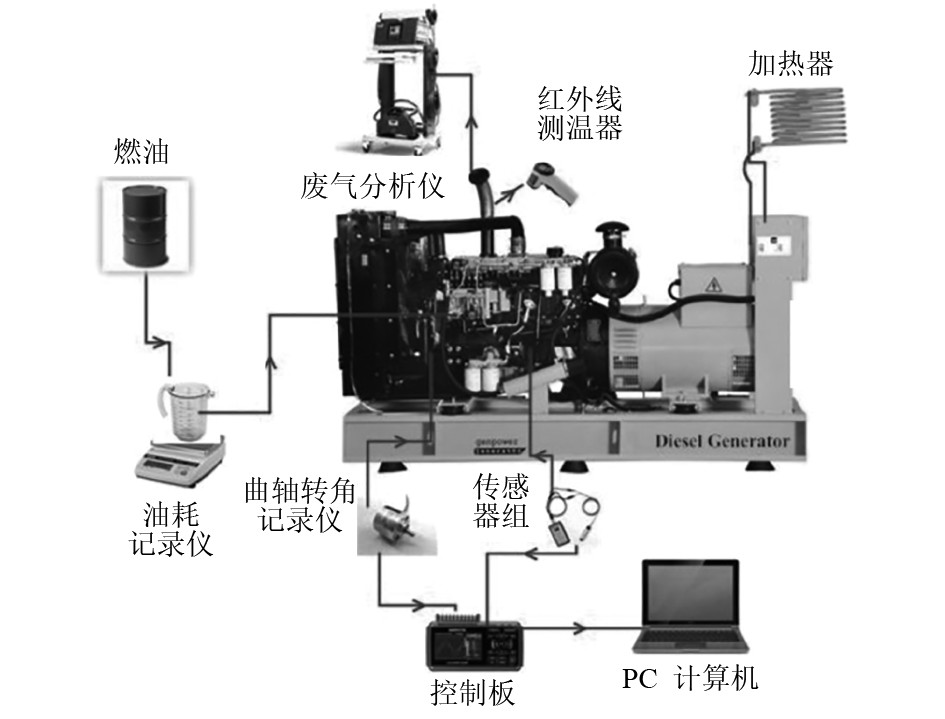图 2 柴油机实验装置简图 Fig. 2 Schematics of experimental device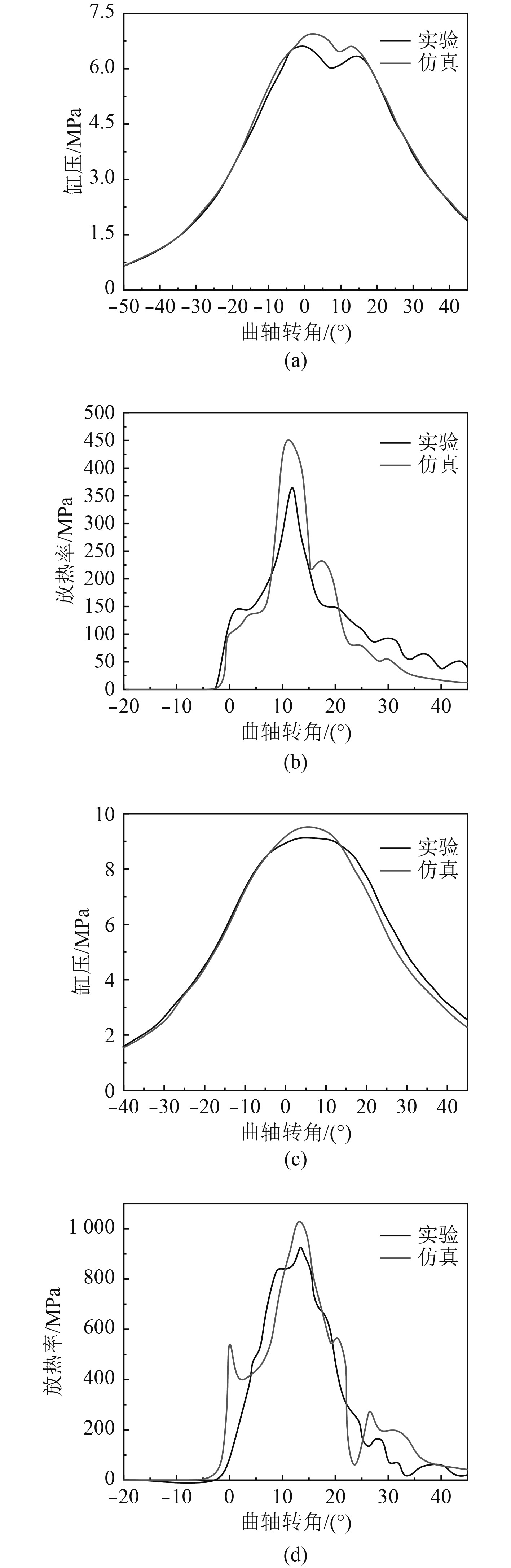图 3 仿真和实验结果对比 Fig. 3 Comparisons of simulation and experiment results

2 仿真结果分析 2.1 柴油机燃烧特性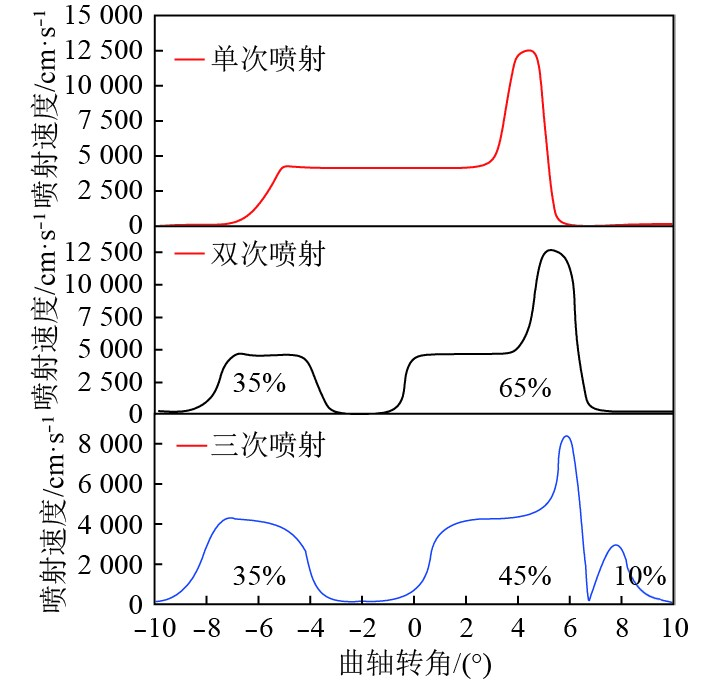图 4 柴油机燃油喷射方案 Fig. 4 Injection profile and injected mass percentages

1）缸压

2种工况下，3种喷油速率仿真的缸压变化如图5所示。从图5（a）可以看出，在柴油机低负荷下，柴油机的缸压出现了2个波峰。虽然缸内燃烧在上止点前就开始了，但是燃烧释放的热量不足以克服由于膨胀减小的压力，即在上止点后，产生了第1个波峰。然而，当剧烈燃烧时，更多释放的热量导致了第2个波峰的出现。因此，可以发现燃油喷射量和喷油持续时间是非常关键的2个因素，如果不能精确地控制两者，还可能会导致发火失败。从图5（b）可以看出，在高负荷时，柴油机的缸压曲线并没有出现2个波峰，而是在上止点10°后出现了一个波峰。这主要是由于高负荷时喷入了较多的燃料，缸内初期燃烧时释放了较多能量。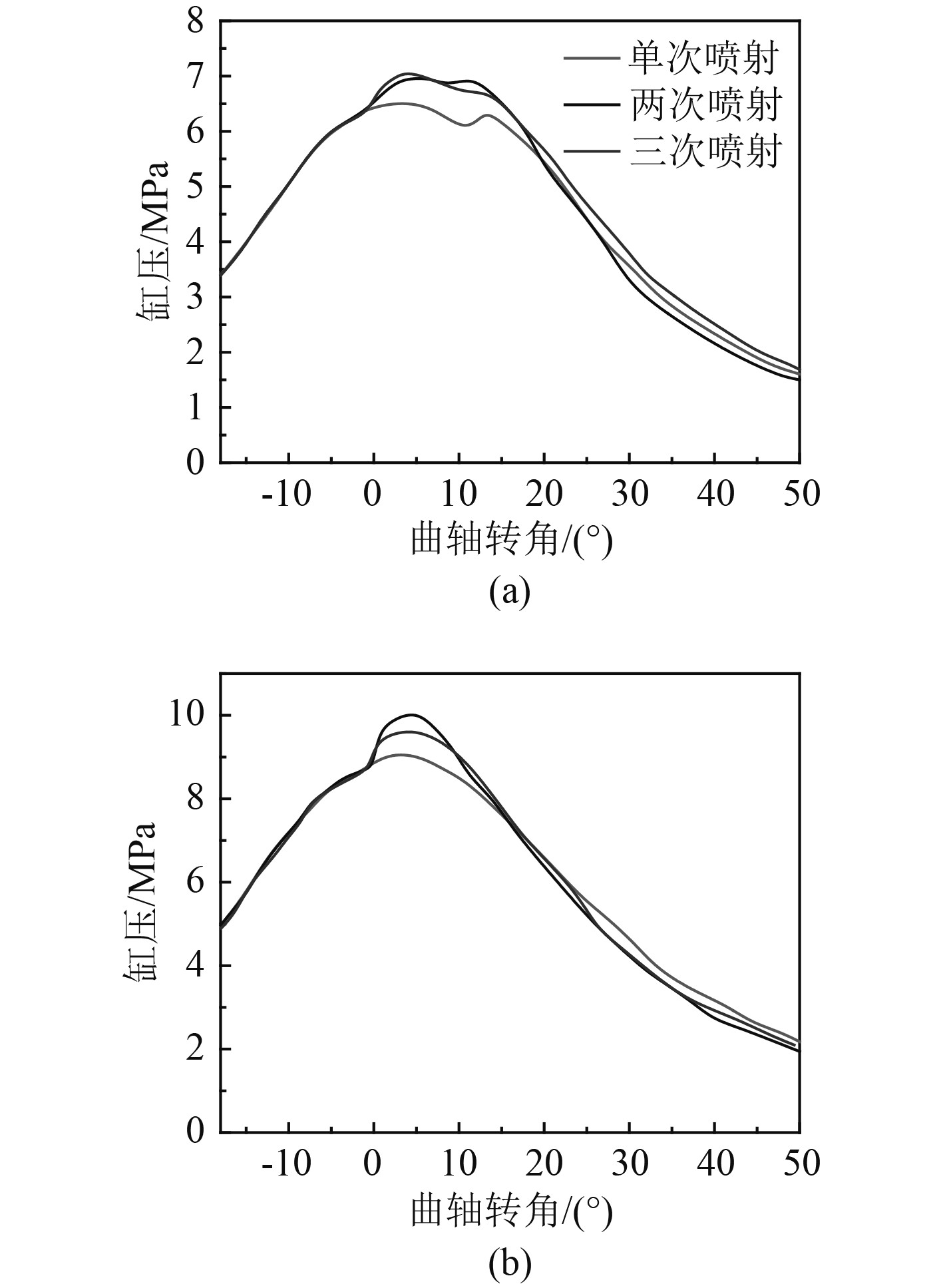图 5 不同工况柴油机缸压变化情况 Fig. 5 Cylinder pressure under different conditions

2）放热率

2种工况下，3种喷油速率仿真的放热率如图6所示。可以清楚地发现，低转速时柴油机的燃烧时刻明显比高转速的燃烧时刻更早。这主要是由于转速较低时燃油和空气的预混合时间和点火延时变长导致。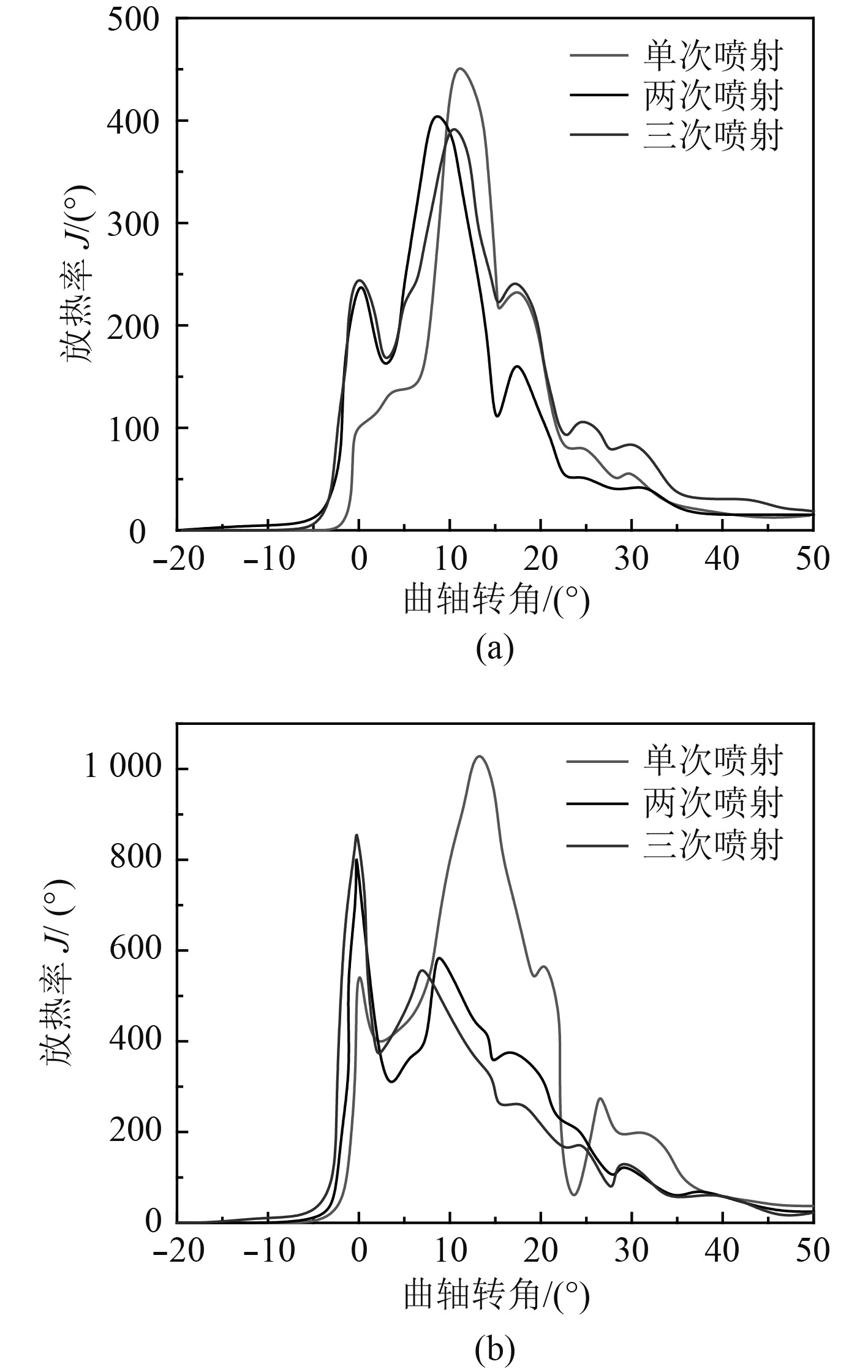图 6 不同工况柴油机放热率变化情况 Fig. 6 Heat release rate under different conditions

3）缸内温度

2种工况下，3种喷油速率仿真的缸内温度如图7所示。从图7（a）可以清楚地发现，在低转速时单次喷射的缸内温度明显低于两次和三次喷射的温度。这主要是由于两次喷射和三次喷射的燃烧时刻早于一次喷射的燃烧，且从图6可得其燃烧明显早于一次喷射。从图7（b）可以看出，高速时，三次喷射的缸内温度最高，其次为两次喷射和三次喷射。这主要是两次和三次喷射的时刻在上止点11°，而一次喷射在上止点9°，其燃烧早于单次喷射。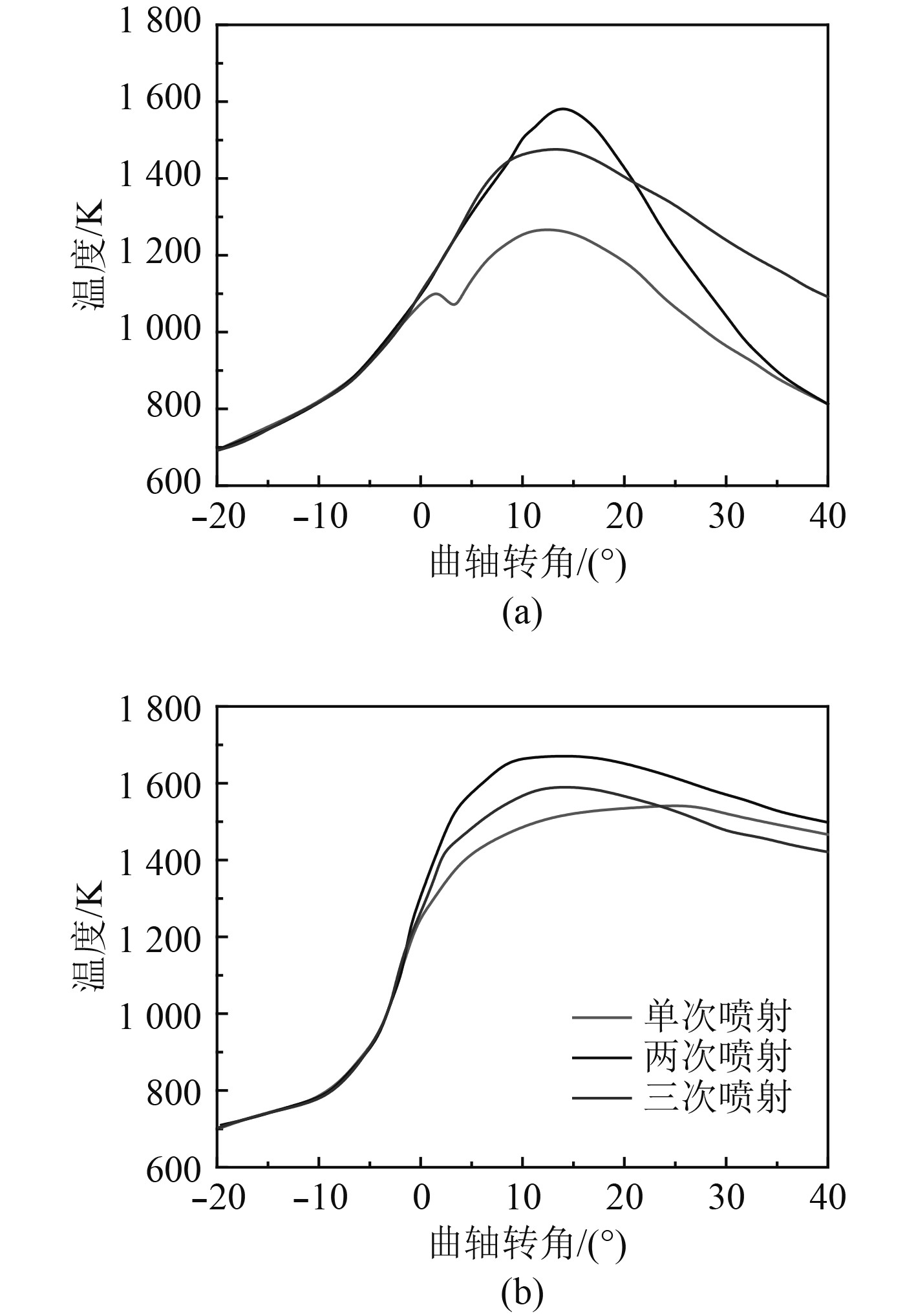图 7 不同工况柴油机缸内温度变化情况 Fig. 7 Cylinder temperature under different conditions
2.2 柴油机排放特性

1）CO排放

CO的生成主要受到混合气体和缸内燃烧温度的影响。2种工况下，3种喷油速率仿真计算的CO排放如图8所示。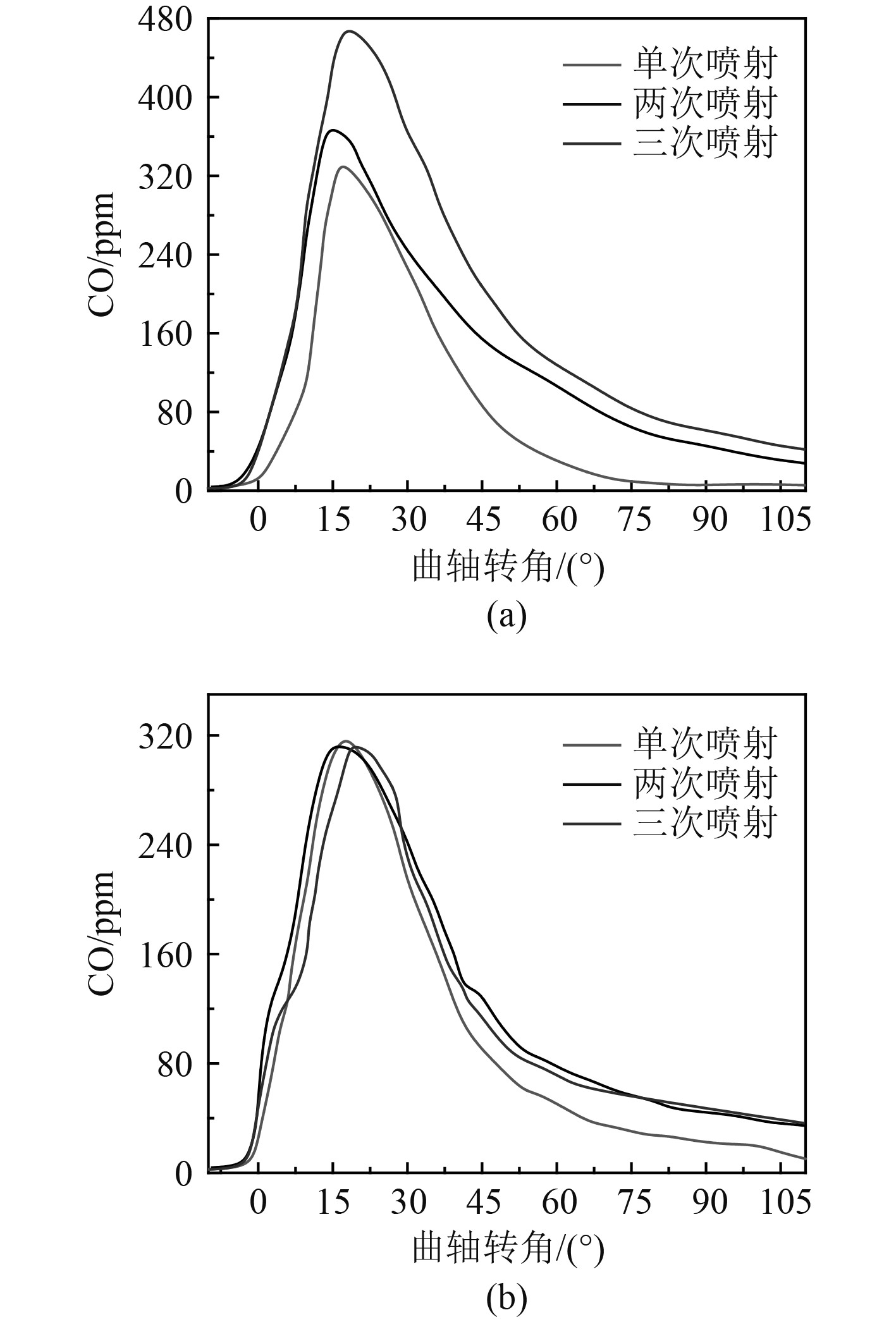图 8 不同工况柴油机CO排放情况 Fig. 8 CO emission under different conditions

2）NOx排放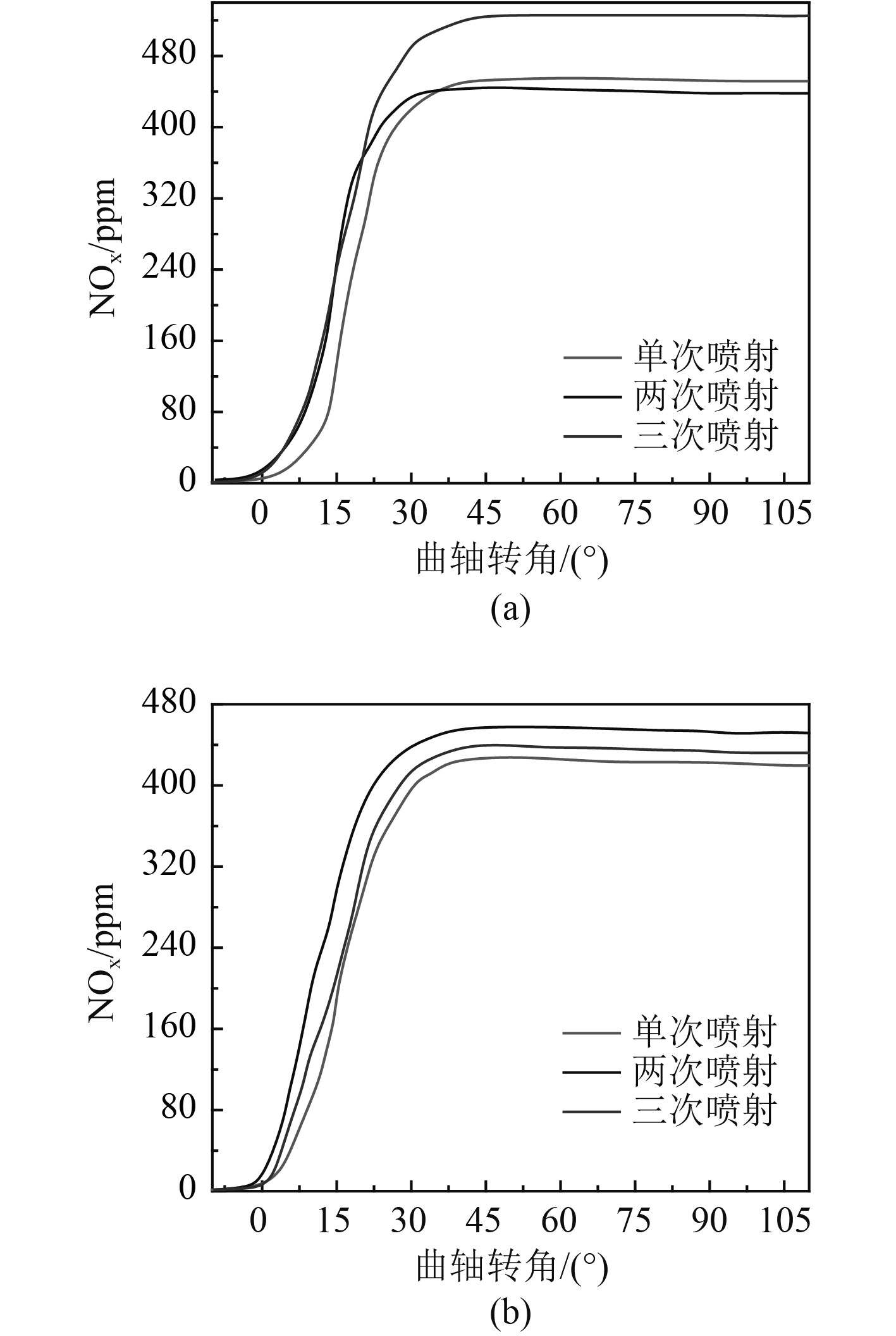图 9 不同工况柴油机NOx排放情况 Fig. 9 NOx emission under different conditions

3 结 　语

  王存磊, 殷承良, 王磊. BOOST发动机建模及其在混合动力仿真中的应用[J]. 上海交通大学学报, 2011, 45(6): 875-879.  朱益梁, 何勇灵. 可变压缩比对增压中冷柴油机性能影响的模拟分析[J]. 农业工程学报, 2012, 28(4): 27-32. DOI:10.3969/j.issn.1002-6819.2012.04.005  LUO ZY, PLOMER M, LU TF, SOM S,et al. A reduced mechanism for biodiesel surrogates for compression ignition engine appli-cations[J]. Fuel, 2012, 99: 143-153. DOI:10.1016/j.fuel.2012.04.028  BIRTAS A, CHIRIAC R. A study of injection timing for a diesel engine operating with gasoil and HRG gas[J]. UPB Scientific Bulletin, Bucharest, 2011.  ZHANG ZQ, E JQ, DENG YW, etal. Effect of fatty acid methyl esters proportion on combustion and emission characteristics of a biodiesel fueled marine diesel engine[J]. Energy Conversion and Management, 2018, 159: 244-253. DOI:10.1016/j.enconman.2017.12.098  FISHER EM, PITZ WJ, CURRAN HJ, etal. Detailed chemical kinetic mechanisms for combustion of oxygenated fuels[J]. Proceeding of the Combustion Institute, 2000, 28: 1579-1586. DOI:10.1016/S0082-0784(00)80555-X  BRAKORA JL, R RD. Investigation of NOx predictions from biodiesel-fueled HCCI engine simulations using a reduced kinetic mechanism[J]. SAE paper, 201001-0577.  NG HK, GAN SY, NG JH,etal. Development and validation of a reduced combined biodiesel-diesel reaction mechanism[J]. Fuel, 2013, 104: 620-634. DOI:10.1016/j.fuel.2012.07.033  SCHWAB AW, BAGBY MO, FREEMAN B. Preparation and properties of diesel fuels from vegetable oils[J]. Fuel, 1987, 66(10): 1372-1378. DOI:10.1016/0016-2361(87)90184-0  KUMAR MS, BELLETTRE J, TAZEROUT M. Investigations on a CI engine using animal fat and its emulsions with water and methanol as fuel[J]SAE Technical Paper, 2005-01-1729.  ZHOU DZ, YANG WM, AN H, etal. A numerical study on RCCI engine fueled by biodiesel/methanol[J]. Energy Conversion and Management, 2015, 89: 798-807. DOI:10.1016/j.enconman.2014.10.054